Courses

# Flexible Pavement Notes | EduRev

## Civil Engineering (CE) : Flexible Pavement Notes | EduRev

The document Flexible Pavement Notes | EduRev is a part of the Civil Engineering (CE) Course Transportation Engineering.
All you need of Civil Engineering (CE) at this link: Civil Engineering (CE)

Introduction

Flexible pavements are those, which on the whole have low or negligible flexural strength and are rather flexible in their structural action under the loads.
A typical flexible pavement consists of four components:

2. sub-base course
3. base course
4. surface course.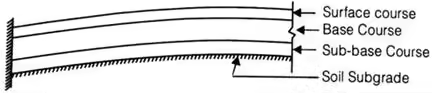(i) Stress Under Road Surface as per Boussineq’s Equation,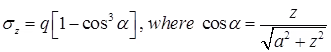where,
σz = vertical stress at depth z.
q = surface pressure.
z = depth at which σz is computed.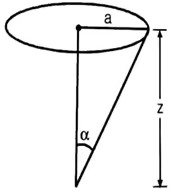(ii) As per IRC
Maximum legal axle load = 8170 kg
Equivalent single wheel load = 4085 kg.
(iii) Contact pressure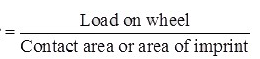(iv) Rigidity factor (R.F)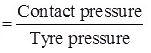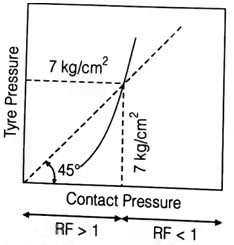(v) Equivalent Single Wheel Load (ESWL)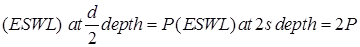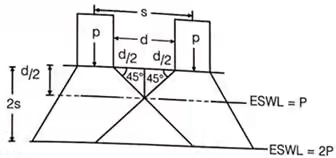Methods of Flexible Pavement Design

(i) Group Index Method
G.I = 0.2a + 0.005ac + 0.01bd

(ii) C.B.R Method
(a)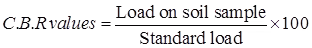(b) The thickness of Pavement, (T)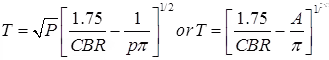where, P = Wheel load in kg.
CBR = California bearing ratio in percent
p = Tyre pressure in kg/cm2
A = Area of contact in cm2.
A = πa2
a = Radius of contact area.

(c) Number of a heavy vehicle per day for design (A),
A = P[1 + r](n + 10)
where, A = No. of vehicles at the end of design period.
P = Number of heavy vehicles per day at least count.
r = Annual rate of increase of heavy vehicles
n = Number of years between the last count & the year of completion of construction.

(d) CBR Method of pavement design by cumulative standard axle load,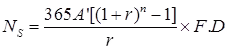where,
Ns = Cumulative number of standard axle load
A’ = Number of the commercial vehicle per day when construction is completed considering the number of lanes.
n = Design life of the pavement, taken as 10 to 15 years.
F = Vehicle damage factor.
D = Lane distribution factor

(iii) California Resistance Value Method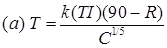where, T = Total thickness of pavement, (cm)
k = Numerical constant = 0.166
T.I = Traffic Index
T.I = 1.35(EWL)0.11
R = Stabilometer resistance value
C = Choesiometer value.where, T1 & T2 are the thickness values of any two pavement layers & C1 & C2 are their corresponding Cohesiometer values.

(iv) Triaxial Method
(a) Thickness of pavement required for single layer, (TS)where, TS = Thickness in cm
P = Wind load in kg
X = Traffic coefficient
Y = Rainfall coefficient
ES = Modulus of elasticity of subgrade soil (kg/cm2)
a = Radius of contact area (cm)
Δ = Design deflection (0.25 cm)

(b) Thickness of Pavement Consist of Two layer system,where, EP = Modulus of elasticity of pavement material
T1/T2 = (ES)/(EP)1/3

T = k.log10(P/S)
where, T = Required thickness of gravel base (cm)
P = Gross wheel load, (kg)
k = Base course constant.

(vi) Burmister Method (Layered System)
Displacement equations given by Burmister are,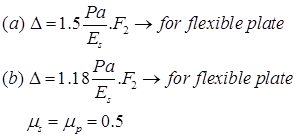where, μs and μp are Poisons ratio for soil subgrade & pavement.
For single layer, F2 = 1
P = Yielded pressure

Offer running on EduRev: Apply code STAYHOME200 to get INR 200 off on our premium plan EduRev Infinity!

## Transportation Engineering

23 videos|66 docs|39 tests

,

,

,

,

,

,

,

,

,

,

,

,

,

,

,

,

,

,

,

,

,

;